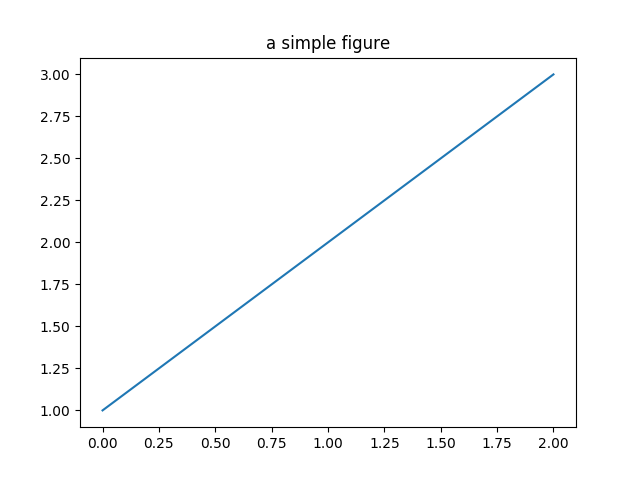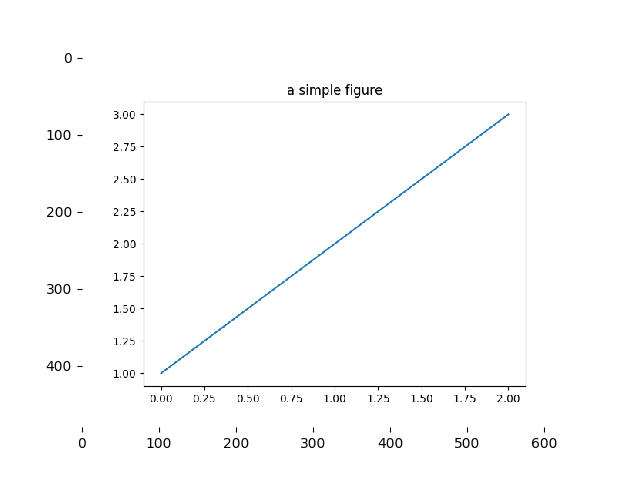# Agg Buffer To Array¶

Convert a rendered figure to its image (NumPy array) representation.

••import matplotlib.pyplot as plt
import numpy as np

# make an agg figure
fig, ax = plt.subplots()
ax.plot([1, 2, 3])
ax.set_title('a simple figure')
fig.canvas.draw()

# grab the pixel buffer and dump it into a numpy array
X = np.array(fig.canvas.renderer._renderer)

# now display the array X as an Axes in a new figure
fig2 = plt.figure()